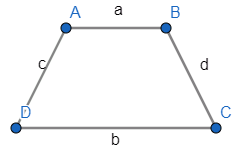QUESTION

# A field is in the shape of a trapezium whose parallel sides are 25m and 10m. If its non-parallel sides are 14m and 13m, find its area.

- Hint: In this question, we first need to draw the diagram with the given conditions. Then by using the formula for area of trapezium on substituting the respective values we can get the result.

Complete step-by-step solution -

TRAPEZIUM:
If one pair of opposite sides of a quadrilateral are parallel, then it is called a trapezium.
Area of a trapezium when the length of the parallel sides and non-parallel sides are given is
$\dfrac{a+b}{k}\sqrt{s\left( s-k \right)\left( s-c \right)\left( s-d \right)}$
Where,
a, b are the parallel sides
c, d are the non-parallel sides
\begin{align} & k=b-a \\ & s=\dfrac{k+c+d}{2} \\ \end{align}
Let us now draw the diagram of the trapezium.Given, in the question that
\begin{align} & a=10 \\ & b=25 \\ & c=14 \\ & d=13 \\ \end{align}
Now, from the above formula k is given by
$\Rightarrow k=b-a$
Now, by substituting the respective values of a and b we get,
\begin{align} & \Rightarrow k=25-10 \\ & \therefore k=15 \\ \end{align}
Let us now find the value of s from the above formula
$\Rightarrow s=\dfrac{k+c+d}{2}$
Now, by substituting the values k, c, d accordingly in the above formula we get,
$\Rightarrow s=\dfrac{15+14+13}{2}$
Now, on further simplification we get,
\begin{align} & \Rightarrow s=\dfrac{42}{2} \\ & \therefore s=21 \\ \end{align}
Let us assume the area of the trapezium as A
Now, by substituting the respective values in the above area of the trapezium formula we get,
$\Rightarrow A=\dfrac{a+b}{k}\sqrt{s\left( s-k \right)\left( s-c \right)\left( s-d \right)}$
Let us now substitute the respective values.
$\Rightarrow A=\dfrac{25+10}{15}\sqrt{21\left( 21-15 \right)\left( 21-14 \right)\left( 21-13 \right)}$
Now, on subtracting the terms under the square root accordingly we get,
$\Rightarrow A=\dfrac{25+10}{15}\sqrt{21\times 6\times 7\times 8}$
Now, on adding and multiplying the respective terms and further simplifying we get,
$\Rightarrow A=\dfrac{35}{15}\sqrt{7056}$
Now, on cancelling the common terms and rewriting the square root term we get,
$\Rightarrow A=\dfrac{7}{3}\sqrt{{{\left( 84 \right)}^{2}}}$
Now, on further simplification we get,
\begin{align} & \Rightarrow A=\dfrac{7}{3}\times 84 \\ & \Rightarrow A=7\times 28 \\ & \therefore A=196{{m}^{2}} \\ \end{align}
Hence, the area of the given trapezium is 196 square metres.

Note: Instead of using the formula for area of trapezium when parallel and non-parallel sides are given we can use the normal formula that includes parallel sides and the height in which we need to find the height by using the non-parallel sides. Both the methods give the same result.
While calculating the area respective terms or side length should be substituted because neglecting any of the terms causes the square root to be unsolvable and then we cannot get the result. It is important to note that b should be greater than a because if not then the value of k will be negative which gives area as negative.